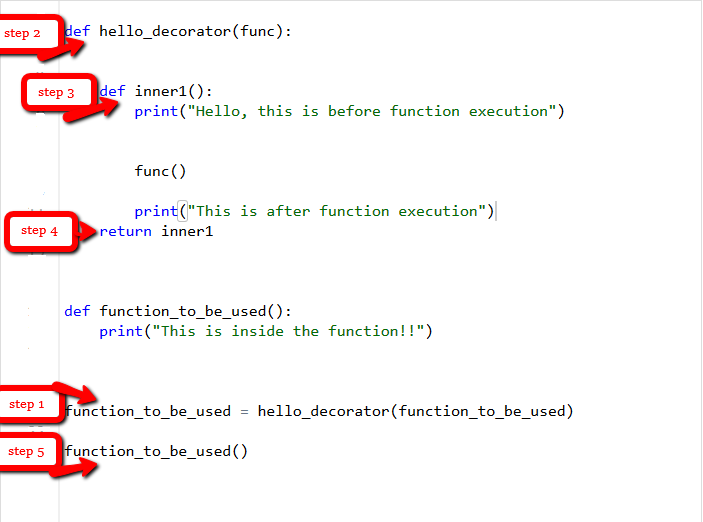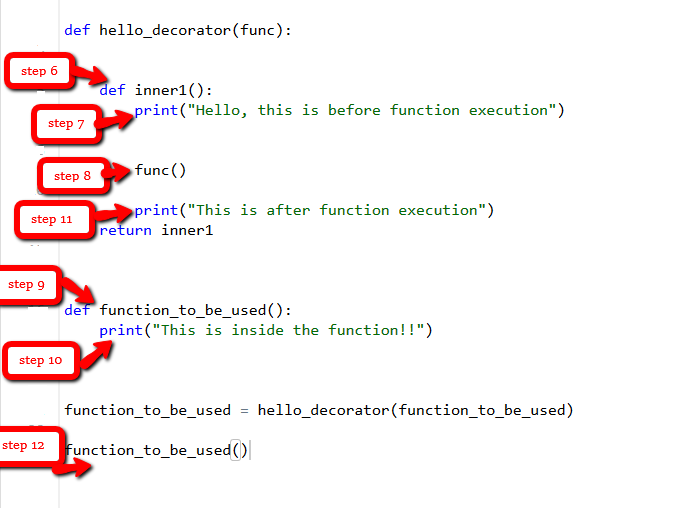Related Articles

# Decorators in Python

• Difficulty Level : Medium
• Last Updated : 01 Mar, 2021

Decorators are very powerful and useful tool in Python since it allows programmers to modify the behavior of function or class. Decorators allow us to wrap another function in order to extend the behavior of the wrapped function, without permanently modifying it. But before diving deep into decorators let us understand some concepts that will come in handy in learning the decorators.

## First Class Objects

In Python, functions are first class objects that means that functions in Python can be used or passed as arguments.

Properties of first class functions:

• A function is an instance of the Object type.
• You can store the function in a variable.
• You can pass the function as a parameter to another function.
• You can return the function from a function.
• You can store them in data structures such as hash tables, lists, …

Consider the below examples for better understanding.

Example 1: Treating the functions as objects.

 `# Python program to illustrate functions ``# can be treated as objects ``def` `shout(text): ``    ``return` `text.upper() `` ` `print``(shout(``'Hello'``)) `` ` `yell ``=` `shout `` ` `print``(yell(``'Hello'``)) `

Output:

```HELLO
HELLO
```

In the above example, we have assigned the function shout to a variable. This will not call the function instead it takes the function object referenced by shout and creates a second name pointing to it, yell.

Example 2: Passing the function as argument

 `# Python program to illustrate functions ``# can be passed as arguments to other functions ``def` `shout(text): ``    ``return` `text.upper() `` ` `def` `whisper(text): ``    ``return` `text.lower() `` ` `def` `greet(func): ``    ``# storing the function in a variable ``    ``greeting ``=` `func(``"""Hi, I am created by a function passed as an argument."""``) ``    ``print` `(greeting) `` ` `greet(shout) ``greet(whisper) `

Output:

```HI, I AM CREATED BY A FUNCTION PASSED AS AN ARGUMENT.
hi, i am created by a function passed as an argument.
```

In the above example, the greet function takes another function as a parameter (shout and whisper in this case). The function passed as argument is then called inside the function greet.

Example 3: Returning functions from another functions.

 `# Python program to illustrate functions ``# Functions can return another function `` ` `def` `create_adder(x): ``    ``def` `adder(y): ``        ``return` `x``+``y `` ` `    ``return` `adder `` ` `add_15 ``=` `create_adder(``15``) `` ` `print``(add_15(``10``)) `

Output:

```25
```

In the above example, we have created a function inside of another function and then have returned the function created inside.

The above three examples depict the important concepts that are needed to understand decorators. After going through them let us now dive deep into decorators.

## Decorators

As stated above the decorators are used to modify the behavior of function or class. In Decorators, functions are taken as the argument into another function and then called inside the wrapper function.

Syntax for Decorator:

```@gfg_decorator
def hello_decorator():
print("Gfg")

'''Above code is equivalent to -

def hello_decorator():
print("Gfg")

hello_decorator = gfg_decorator(hello_decorator)'''
```

In the above code, `gfg_decorator` is a callable function, will add some code on the top of some another callable function, `hello_decorator` function and return the wrapper function.

Decorator can modify the behavior:

 `# defining a decorator``def` `hello_decorator(func):`` ` `    ``# inner1 is a Wrapper function in ``    ``# which the argument is called``     ` `    ``# inner function can access the outer local``    ``# functions like in this case "func"``    ``def` `inner1():``        ``print``(``"Hello, this is before function execution"``)`` ` `        ``# calling the actual function now``        ``# inside the wrapper function.``        ``func()`` ` `        ``print``(``"This is after function execution"``)``         ` `    ``return` `inner1`` ` ` ` `# defining a function, to be called inside wrapper``def` `function_to_be_used():``    ``print``(``"This is inside the function !!"``)`` ` ` ` `# passing 'function_to_be_used' inside the``# decorator to control its behavior``function_to_be_used ``=` `hello_decorator(function_to_be_used)`` ` ` ` `# calling the function``function_to_be_used()`

Output:

```Hello, this is before function execution
This is inside the function !!
This is after function execution```

Let’s see the behavior of the above code how it runs step by step when the “function_to_be_used” is called.Let’s jump to another example where we can easily find out the execution time of a function using a decorator.

 `# importing libraries``import` `time``import` `math`` ` `# decorator to calculate duration``# taken by any function.``def` `calculate_time(func):``     ` `    ``# added arguments inside the inner1,``    ``# if function takes any arguments,``    ``# can be added like this.``    ``def` `inner1(``*``args, ``*``*``kwargs):`` ` `        ``# storing time before function execution``        ``begin ``=` `time.time()``         ` `        ``func(``*``args, ``*``*``kwargs)`` ` `        ``# storing time after function execution``        ``end ``=` `time.time()``        ``print``(``"Total time taken in : "``, func.__name__, end ``-` `begin)`` ` `    ``return` `inner1`` ` ` ` ` ` `# this can be added to any function present,``# in this case to calculate a factorial``@calculate_time``def` `factorial(num):`` ` `    ``# sleep 2 seconds because it takes very less time``    ``# so that you can see the actual difference``    ``time.sleep(``2``)``    ``print``(math.factorial(num))`` ` `# calling the function.``factorial(``10``)`

Output:

```3628800
Total time taken in :  factorial 2.0061802864074707```

## What if a function returns something or an argument is passed to the function?

In all the above examples the functions didn’t return anything so there wasn’t any issue, but one may need the returned value.

 `def` `hello_decorator(func):``    ``def` `inner1(``*``args, ``*``*``kwargs):``         ` `        ``print``(``"before Execution"``)``         ` `        ``# getting the returned value``        ``returned_value ``=` `func(``*``args, ``*``*``kwargs)``        ``print``(``"after Execution"``)``         ` `        ``# returning the value to the original frame``        ``return` `returned_value``         ` `    ``return` `inner1`` ` ` ` `# adding decorator to the function``@hello_decorator``def` `sum_two_numbers(a, b):``    ``print``(``"Inside the function"``)``    ``return` `a ``+` `b`` ` `a, b ``=` `1``, ``2`` ` `# getting the value through return of the function``print``(``"Sum ="``, sum_two_numbers(a, b))`

Output:

```before Execution
Inside the function
after Execution
Sum = 3```

In the above example, you may notice a keen difference in the parameters of the inner function. The inner function takes the argument as *args and **kwargs which means that a tuple of positional arguments or a dictionary of keyword arguments can be passed of any length. This makes it a general decorator that can decorate a function having any number of arguments.

## Chaining Decorators

In simpler terms chaining decorators means decorating a function with multiple decorators.

Example:

 `# code for testing decorator chaining ``def` `decor1(func): ``    ``def` `inner(): ``        ``x ``=` `func() ``        ``return` `x ``*` `x ``    ``return` `inner `` ` `def` `decor(func): ``    ``def` `inner(): ``        ``x ``=` `func() ``        ``return` `2` `*` `x ``    ``return` `inner `` ` `@decor1``@decor``def` `num(): ``    ``return` `10`` ` `print``(num()) `

Output:

```400
```

The above example is similar to calling the function as –

```decor1(decor(num))
```

Attention geek! Strengthen your foundations with the Python Programming Foundation Course and learn the basics.

To begin with, your interview preparations Enhance your Data Structures concepts with the Python DS Course. And to begin with your Machine Learning Journey, join the Machine Learning – Basic Level Course

My Personal Notes arrow_drop_up